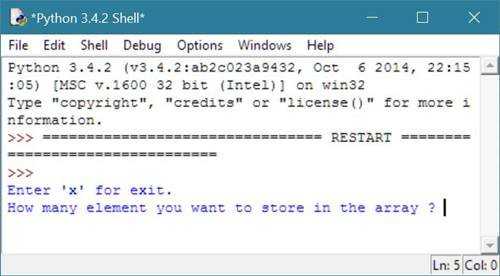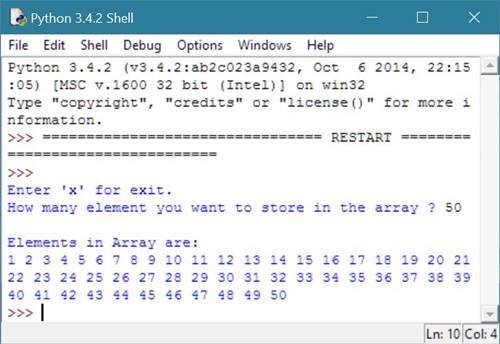# Python One Dimensional Array Program

## One Dimensional Array Program in Python

To perform one dimensional array in python, follow the program given below. This program illustrates how to use one dimensional array in python.

## Python Programming Code to One Dimensional Array

Following python program ask from user to enter the total number of elements, he/she wants to store in the array. And then store all the elements in the array. The value of the element starts from 1 to the number of term he/she entered:

```# Python Program - One Dimensional Array

print("Enter 'x' for exit.");
nval = input("How many element you want to store in the array ? ");
if nval == 'x':
exit();
else:
n = int(nval);
arr = [];
for i in range(1, n+1):
arr.append(i);
print("\nElements in Array are:");
for i in range(0, n):
print(arr[i], end=" ");
```

Here is the sample run of the above python program to illustrate one dimensional array:Now enter total number of element say 50 and press enter key to see all the elements stored in an array from 1 to 50 as shown here in below sample output:Below is the same program on python shell:### Same Program in Other Languages

You may also like to learn or practice the same program in other popular programming languages:

Tools
Calculator

Quick Links
Signup - Login - Give Online Test